# Simply-supported Sandwich PlateThe purpose of this test is to check the elements on a non-isotropic plate structure. Transverse shear effects are necessary, but Kirchoff elements must not be used.

The reference is the mesh formed of parabolic hexahedron elements (HE20).
You will use a 2D meshes.Reference:

Modélisation des structures par éléments finis, BATOZ J.L., DHATT G., Vol.2, p.341; Hermès Edition, Paris 1990.

##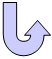Specifications

### Geometry Specifications

 Length: L = 100 mm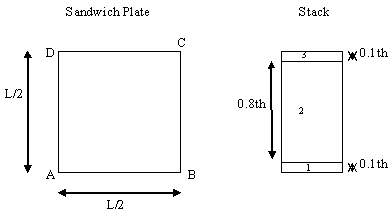Thickness: th = 10 mm Sandwich: 0/0/0 deg

### Analysis Specifications

 Face Sheets: Young Modulus (material): E11 = 341.56 MPa E22 = 179.3 MPa Shear Modulus: G12 = 100 MPa G13 = 60.8 MPa G23 = 101.5 MPa Poisson's Ratio: ν = 0.44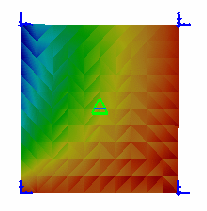Transverse shear stress Core: Young Modulus (material): E11 = 34.156 MPa E22 = 17.93 MPa Shear Modulus: G12 = 10.0 MPa G13 = 6.08 MPa G23 = 10.15 MPa Poisson's Ratio: ν12 = 0.44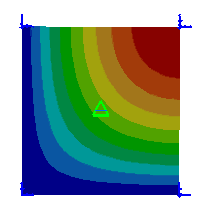Translational Displacement Mesh Specifications: Size of elements = 5 mm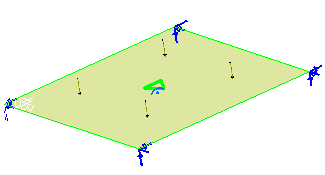Load and restraints Restraints (User defined): Edge AB: Tx = Ty = Tz = Ry = 0 Edge BC: Tx = Ty =  Ry = Rz= 0 Edge CD: Tx = Ty = Rx = Rz = 0 Edge AD: Tx = Ty = Tz = Rx = 0 Loads: Pressure P = 1e+006 N_m2

##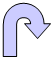Results

The results correspond to:

• the displacement in the z-direction at point C
• the stress σxx at point C in the middle of the upper layer
• the stress σxz at point D in the middle of the core.

The computed results are given in the table below.

 Quantity Point of localization Reference Solution: Parabolic Hexahedron (HE20) Linear Triangle (TR3) Parabolic Triangle (TR6) Linear Quadrangle (QD4) Parabolic Quadrangle (QD8)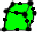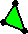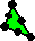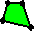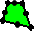W [mm] Point C 41.83 41.088 41.306 42.072 41.987 σxx [MPa] Point C 57.17 57.357 57.964 56.753 56.984 σxz [MPa] Point D 4.128 4.08 4.64 4.04 4.32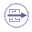To Perform the Test:

The simply_supported_sandwich_plate_he20.CATAnalysis document presents the complete analysis of this case, computed with a mesh formed of parabolic hexahedron elements (HE20). These results are the reference.

The simply_supported_sandwich_plate_tr6.CATAnalysis document presents a complete analysis on an orthotropic analog plate, computed with a mesh formed of parabolic triangle elements (TR6).

To compute the case with other meshes, proceed as follow:

1. Open the simply_supported_sandwich_plate_tr6.CATAnalysis document.

2. In the Advanced Meshing Tools workbench, replace the mesh specifications.

3. In the Generative Structural Analysis workbench, compute the case.